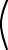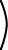IA = A

Identity Matrix

The matrix I is called an identity matrix because IA = A and AI = A for all matrices A.

This is similar to the real number 1, which is called the multiplicative identity, because 1a = a and a1 = a for all real numbers a.

There is no matrix that works as an identity for matrices of all dimensions. For N×N square matrices there is a matrix IN×N that works as an identity. For example, the 3×3 identity is:1 0 0 0 1 0 0 0 1The N-dimensional identity matrix IN×N has 1's on the main diagonal and 0's elsewhere.

The main diagonal consists of the elements with the row number equal to the column number. The 1s must run down that particular diagonal; it won't work with the other diagonal.

QUESTION 4:

Is there another N×N matrix that works like I?